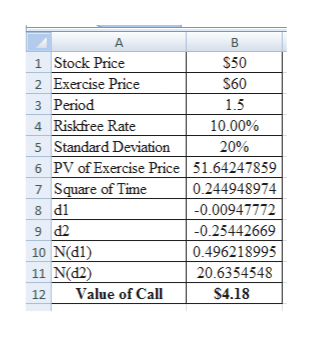# Question 2. (a) Use the Black-Scholes formula to find the current price of a European call option on a stock paying no income with strike 60 and maturity 18 months from now. Assume the current stock price is 50, the lognormal volatility of the stock is σ = 20%, and the constant continuously compounded interest rate is r = 10%.

Question
138 views

Question 2. (a) Use the Black-Scholes formula to find the current price of a European call option on a stock paying no income with strike 60 and maturity 18 months from now. Assume the current stock price is 50, the lognormal volatility of the stock is σ = 20%, and the constant continuously compounded interest rate is r = 10%.

check_circle

Step 1

Calculation of Price of European Call Option:

The price of European call option is \$4.18.

...help_outlineImage TranscriptioncloseA B 1 Stock Price \$50 2 Exercise Price \$60 3 Period 1.5 4 Riskfree Rate 10.00% 5 Standard Deviation 20% 6 PV of Exercise Price 51.64247859 7 Square of Time 0.244948974 -0.00947772 8 d1 -0.25442669 9 d2 0.496218995 10 N(dl) 11 N(d2) 20.6354548 Value of Call \$4.18 12 fullscreen

### Want to see the full answer?

See Solution

#### Want to see this answer and more?

Solutions are written by subject experts who are available 24/7. Questions are typically answered within 1 hour.*

See Solution
*Response times may vary by subject and question.
Tagged in